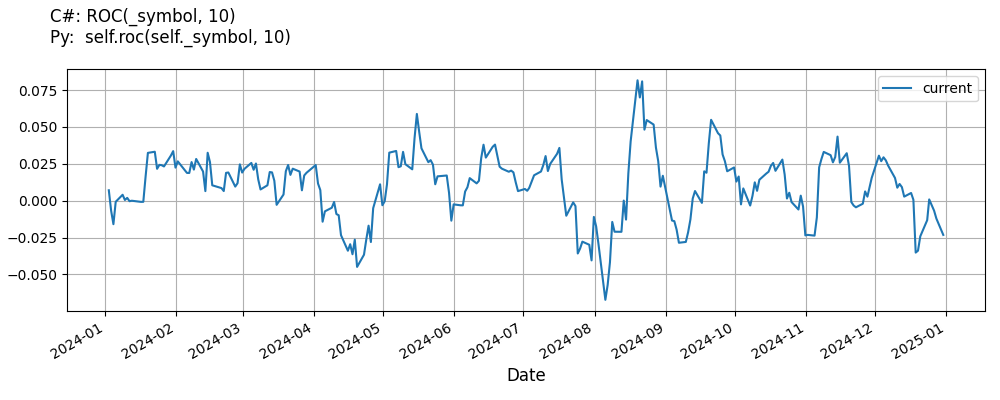# Supported Indicators

## Rate Of Change

### Introduction

This indicator computes the n-period rate of change in a value using the following: (value_0 - value_n) / value_n

To view the implementation of this indicator, see the LEAN GitHub repository.

### Using ROC Indicator

To create an automatic indicators for RateOfChange, call the ROC helper method from the QCAlgorithm class. The ROC method creates a RateOfChange object, hooks it up for automatic updates, and returns it so you can used it in your algorithm. In most cases, you should call the helper method in the Initialize method.

public class RateOfChangeAlgorithm : QCAlgorithm
{
private Symbol _symbol;
private RateOfChange _roc;

public override void Initialize()
{
_roc = ROC(_symbol, 10);
}

public override void OnData(Slice data)
{
{
// The current value of _roc is represented by itself (_roc)
// or _roc.Current.Value
Plot("RateOfChange", "roc", _roc);

}
}
}
class RateOfChangeAlgorithm(QCAlgorithm):
def Initialize(self) -> None:
self.roc = self.ROC(self.symbol, 10)

def OnData(self, slice: Slice) -> None:
# The current value of self.roc is represented by self.roc.Current.Value
self.Plot("RateOfChange", "roc", self.roc.Current.Value)



The following reference table describes the ROC method:

### ROC()1/1

            RateOfChange QuantConnect.Algorithm.QCAlgorithm.ROC (
Symbol                           symbol,
Int32                            period,
*Nullable<Resolution>      resolution,
*Func<IBaseData, Decimal>  selector
)


Creates a new RateOfChange indicator. This will compute the n-period rate of change in the security. The indicator will be automatically updated on the given resolution.

If you don't provide a resolution, it defaults to the security resolution. If you provide a resolution, it must be greater than or equal to the resolution of the security. For instance, if you subscribe to hourly data for a security, you should update its indicator with data that spans 1 hour or longer.

You can manually create a RateOfChange indicator, so it doesn't automatically update. Manual indicators let you update their values with any data you choose.

Updating your indicator manually enables you to control when the indicator is updated and what data you use to update it. To manually update the indicator, call the Update method with time/number pair, or an IndicatorDataPoint. The indicator will only be ready after you prime it with enough data.

public class RateOfChangeAlgorithm : QCAlgorithm
{
private Symbol _symbol;
private RateOfChange _roc;

public override void Initialize()
{
_roc = new RateOfChange(10);
}

public override void OnData(Slice data)
{
if (data.Bars.TryGeValue(_symbol, out var bar))
{
_roc.Update(bar.EndTime, bar.Close);
}

{
// The current value of _roc is represented by itself (_roc)
// or _roc.Current.Value
Plot("RateOfChange", "roc", _roc);

}
}
}
class RateOfChangeAlgorithm(QCAlgorithm):
def Initialize(self) -> None:
self.roc = RateOfChange(10)

def OnData(self, slice: Slice) -> None:
bar = slice.Bars.get(self.symbol)
if bar:
self.roc.Update(bar.EndTime, bar.Close)

# The current value of self.roc is represented by self.roc.Current.Value
self.Plot("RateOfChange", "roc", self.roc.Current.Value)



To register a manual indicator for automatic updates with the security data, call the RegisterIndicator method.

public class RateOfChangeAlgorithm : QCAlgorithm
{
private Symbol _symbol;
private RateOfChange _roc;

public override void Initialize()
{
_roc = new RateOfChange(10);
RegisterIndicator(_symbol, _roc, Resolution.Daily);
}

public override void OnData(Slice data)
{
{
// The current value of _roc is represented by itself (_roc)
// or _roc.Current.Value
Plot("RateOfChange", "roc", _roc);

}
}
}
class RateOfChangeAlgorithm(QCAlgorithm):
def Initialize(self) -> None:
self.roc = RateOfChange(10)
self.RegisterIndicator(self.symbol, self.roc, Resolution.Daily)

def OnData(self, slice: Slice) -> None:
# The current value of self.roc is represented by self.roc.Current.Value
self.Plot("RateOfChange", "roc", self.roc.Current.Value)



The following reference table describes the RateOfChange constructor:

### RateOfChange()1/2

            RateOfChange QuantConnect.Indicators.RateOfChange (
int  period
)


Creates a new RateOfChange indicator with the specified period.

### RateOfChange()2/2

            RateOfChange QuantConnect.Indicators.RateOfChange (
string  name,
int     period
)


Creates a new RateOfChange indicator with the specified period.

### Visualization

The following image shows plot values of selected properties of RateOfChange using the plotly library.You can also see our Videos. You can also get in touch with us via Discord.